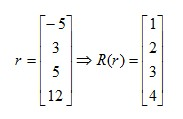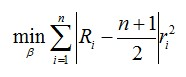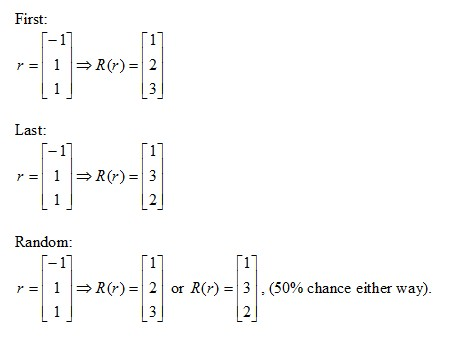# R-estimators

Statistical Analysis Techniques, Robust Estimators, Alternatives to OLS

The three main classes of robust estimators are M, L and R.  Robust estimators are resistant to outliers and when used in regression modelling, are robust to departures from the normality assumption.

R-estimators involve ranking residuals.  The rank of a sample is a mapping from n real numbers to the integers 1 through to n.  The smallest number is given rank 1, the next smallest is given rank 2 etc.  For example:The ranks are used to calculate weights.  For example:When generating an R vector, ties need to be taken into account.  A tie is when two values getting ranked are equal.  For the weights to work,must equal zero.  Several rules for ties can be used to keep this expression true.  They include:### Jaeckel’s estimator

This was proposed by Jaeckel in 1972.  It involves finding the beta coefficient that minimises D:He found that D is non-negative and beta is asymptotically normal with mean β.